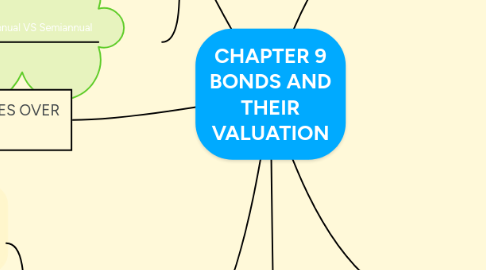# CHAPTER 9 BONDS AND THEIR VALUATION

Get Started. It's FreeCHAPTER 9 BONDS AND THEIR VALUATION## 3. BOND VALUATION

### 3.1. (Par Bond)

3.1.1. rd = coupon rate, fixed-rate bond sells at par

### 3.2. (Discount Bond)

3.2.1. rd > coupon rate, fixed-rate bond sells below par

3.3.1. rd < coupon rate, fixed-rate bond sells above par

## 4. BOND YIELDS

### 4.1. Yield to Maturity

4.1.1. the rate of return earned on a bond if it is held to maturity

### 4.2. Yield to Call

4.2.1. the rate of return earned on a bond when it is called before its maturity date.

4.2.2.1. In general, if a bond sells at a premium, then

4.2.2.1.1. 1) coupon > rd

4.2.2.1.2. 2) a call is more likely

4.2.3. -> Sells at Discount

4.2.4. Expected to Earned

4.2.4.2. YTM on par and discount bonds

## 5. SEMIANNUAL BOND

### 5.1. STEPS :)

5.1.1. 1) Multiply years by 2 : number of periods = 2n.

5.1.2. 2) Divide nominal rate by 2 : periodic rate (I/YR) = rd / 2.

5.1.3. 3) Divide annual coupon by 2 : PMT = ann cpn / 2.

### 5.2. Annual VS Semiannual

5.2.1. (Semiannual effective rate) > (the annual bond’s effective rate)

5.2.1.1. so you would prefer the semiannual bond.